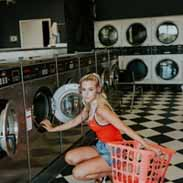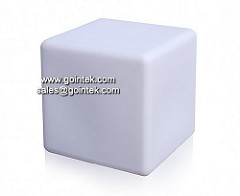# Physical Chemistry Unit 1 ExamRuth Blanco
question

Thermodynamics

Study of the movement of energy; energy moves either through heat or work, or both
question

Heat

Movement of energy is chaotic, and is going all different directions
question

Work

Movement of energy that is direted motion; force; all the movement is going in one direction
question

Classical Thermodynamics

This is the study of thermodynamics that is only concerned with macrospoic properties, microscopic properties of materials (internal structure/atoms) don’t matter ( most of the time)
question

System

Part of the universe we are interested in; visualized as the front face of this particular cube Ex: Liquid in a beaker, gas in a piston
question

SurroundingsRest of the universe apart from what we are interested in; outside of the cube completely
question

BarrierAssociated with the system and surroundings; rests between them; represented visually by the side face of the cube (R)
question

Open Barrier

A barrier between the system and surroundings that allows for exchange of matter and energy Ex: Uncovered beaker
question

Closed Barrier

A barrier between the system and surroudnings that allows for exchange of energy but NOT matter, contains two subcategories
question

A closed barrier that allows for energy to be exchanged btw the system and surroundings but ONLY through work Ex: Piston acting on gas in a container
question

Diathermic Barrier

A closed barrier that allows for energy to be exchanged btw the system and surroundings but ONLY through heat; Ex: test tube combustive reaction surrounded by a water bath at room temp
question

Isolated Barrier

A barrier that does NOT allow energy nor matter to pass; Ex: Thermos
question

Dynamic Equilibrium (Equilibrium)

Keeps the concentration of chemical molecules and the rates of movement equal; however, the molecules of the chemicals DO keep moving; constant diffusion
question

State Variables

Variables that depend on and describe the current state of the system; Ex: Volume, Pressure, Temperature
question

State Equation

An equation that consists ONLY of state variables and a constant Ex: Ideal Gas Law: PV=nRT
question

Process (“Path”) Variables

Variables that depend ONLY on the path taken by the system between equilibrium states; may (and often do) have different values at the same equilbrium state of a system; Ex: work, heat
question

Example of Process (“Path”) VariablesIf the left corner is labeled point A, and an imaginary car drives around and stops at each vertex along the way, when the car returns to A the odometer will read 4 miles even though we are back in the initial position; whereas the GPS would read 0 miles away from home before you leave A and when you return to A
question

Temperature

Indicates the direction of heat flow
question

Thermal Equilibrium

Two objects temperatures do not change when they are brought into contact with each other
question

Movement of Heat

Heat will work on a gradient similar to that of a concentration and electrochemical gradient; heat will transfer from a hot object to a cold object until their temps are in equilibrium
question

0th Law of Thermodynamics

If A and B are in equilibrium, and B and C are in equilibrium, then A and C are also in equilibrium
question

Applications of the 0th Law of Thermodynamics

C allows for us to determine the temperature of A+B ; temperature exists and it can be measured
question

Perfect Gases

Described by the ideal gas law; gas mlcs are in constant and random motion (diminished or not present at all if attractive/repulsive forces are acting on the mlcs); mlcs act as point masses (take up no volume in space); collisions btw mlcs/wall are perfectly elastic (equal force and energy upon entrance and exit from collision)
question

Real Gases

Gases do not behave in an ideal manner due to attractive and repulsive forces; includes IMF (London dispersion forces, dipole-dipole, H-bonding)
question

Real Gases and Forces

*Attractive forces are much more significant and travel over longer distances, than the repulsive forces that affect the behavior fo the gasses*
question

Repulsive Forces

Volume that is taken up by the gas molecules itself; partial/full charges and their interactions
question

Real Gas Deviation

As real gasses approach higher and higher pressures, there is further deviation from the ideal gas laws
question

Attractive Forces Consequence

When these forces are acting on a gas, they promote compression of the gas
question

Repulsive Forces Consequence

When these forces are acting on a gas, they promote expansion of the gas
question

Attractive and Repulsive Forces

These forces both act over short distances within and btw the gas mlcs, but they can be ignored when the pressure is low
question

Virial Eqn

Modifies the ideal gas law by adding a power series incorporating experimentally derived constants; very accurate, but finding the appropriate coefficients at the specific temperature is difficult
question

Van der Waals eqn

Adds two coefficients to account for attractive and repulsive forces; more general and less precise than the Virial eqn; b represents repulsion, and a represents attraction; *CANNOT use extreme values for pressure, temperature, and volume*, a and b are indpendent from other variables
question

Chemical process that occurs at constant heat
question

Isothermal Process

Chemical Process that occurs at constant temperature
question

Isobaric Process

Chemical process that occurs at constant pressure
question

Isochoric Process

Chemical process that occurs at constant volume
question

Isenthalpic Process

Chemical process that occurs at constant enthalpy (delta H)
question

Isentropic Process

Chemical process that occurs at constant entropy (delta S)
question

Internal Energy

Sum of all potential and kinetic energy internal to the system; state function; U=q+w
question

First Law of Thermodynamics

Internal energy of an isolated system is constant; can be thought of as a law of conservation of internal energy (Can’t add more energy to the system or take energy away from the system, the energy just changes while remaining in equilibrium)
question

Expansion Work

Work arising from a change in volume; dw=-pdV
question

Reversible Processes

We assume these processes behave as though they are in equilibrium; consider this is true at every point along the path of the process; Does the maximum work
question

Constant Volume Heat Capacity

Change in internal energy with respect to temperature at a constant volume
question

Constant Pressure Heat Capacity

Change in internal energy with respect to temperature at a constant pressure
question

Enthalpy

Energy supplied to a system as heat at constant pressure; state function: H=U+pV; can be measured using calorimetry (delta H=q (constant pressure))
question

Standard Enthalpies

Standard state of a substance is its pure form at the pressure of one bar and specified temperature (typically 298K)
question

Enthalpy Changes